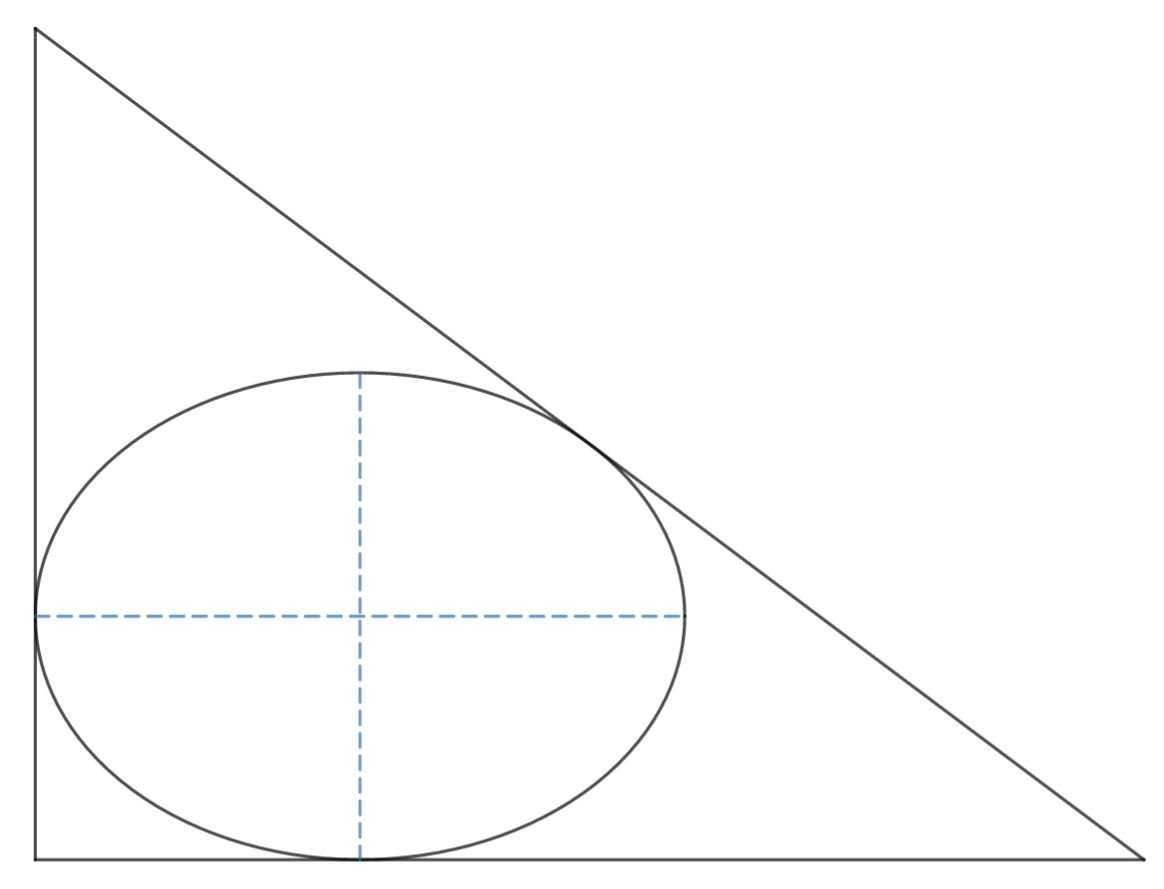# Highly Elliptical AreaAn ellipse is inscribed in a right triangle such that its major and minor axes are parallel to the perpendicular legs of the right triangle.

If the area of the ellipse is $A_1$, the area of the right triangle is $A_2$, and the maximum value of $\dfrac {A_1}{A_2}$ is $\pi (a - \sqrt b)$, where $a$ and $b$ are positive integers, find $a^2+b^2$.

Try similar problems

All of my problems are original.

×